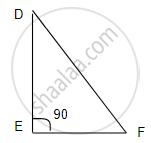# In δDef, If ∠E = 90°, Then Find the Value of ∠D + ∠F. - Geometry

Sum

In ΔDEF, if ∠E = 90°, then find the value of ∠D + ∠F.

#### Solution

In ΔDEFSum of all angle of Triangle = 180°

∠D + ∠E + ∠F = 180°

∠D + 90° + ∠F = 180°

∠D + ∠F  = 180° - 90°

∠D + ∠F  = 90°

Concept: Property of 30°- 60°- 90° Triangle Theorem
Is there an error in this question or solution?